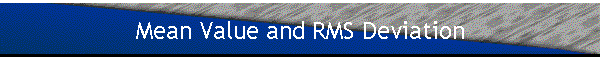The mean value in Quantum Mechanics is the average result obtained when a large number of measurements are made on identical systems, i.e. systems in the same state |Ψ(t)>.
For the harmonic oscillator
<X> = <Φn|X|Φn> = 0,  <P> = <Φn|P|Φn> = 0.
The mean value of X and P in an eigenstate of H is always zero.

The classical mean value can be defined as the average result obtained when a large number of measurements are made on the same system at different times, or when a large number of measurements are made at the same time on different systems, whose phases are randomly chosen.
xavg = (xM/T)∫0Tcos(ωt + φ)dt = 0,  T = 2π/ω = period,
or
xavg = (xM/2π)∫0cos(ωt + φ)dφ = 0.
pavg = -mv = -(mωxM/T)∫0Tsin(ωt + φ)dt = 0,  or  pavg = -(mωxM/2π)∫0sin(ωt + φ)dφ = 0.

For a harmonic oscillator of well-defined energy <X> = xavg = 0,  <P> = pavg = 0.
But we have to remember that the classical and quantum mechanical mean value are interpreted differently.

In a similar way the quantum mechanical and the classical root-mean-square deviation are interpreted differently.
In Quantum Mechanics we define
(ΔX)2 = <X2> - <X>2 = <X2>,  (ΔP)2 = <P2> - <P>2 = <P2>.
X2 = (ħ/(2mω))(a + a)(a + a) = (ħ/(2mω))(aa + aa + aa + aa),
P2 = -(mħω/2)(a - a)(a - a) = (mħω/2)(-aa + aa + aa - aa).
n|aan> = <aΦn|aΦn> ∝ <Φn-1n+1> = 0.
n|aa|Φn> = <aΦn|aΦn> ∝ <Φn+1n-1> = 0.
n|aa|Φn> = <aΦn|aΦn> = n.
n|aan> = <aΦn|aΦn> = n + 1.
<X2> = (ħ/(2mω))(2n + 1) = (ħ/(mω))(n + ½).
<P2> = (mħω/2)(2n + 1) = mħω(n + ½).
ΔXΔP = (n + ½)ħ.
ΔXΔP = ½ħ for n = 0, i.e. for the ground state.

For a classical harmonic oscillator with energy E = (n + ½)ħω we have
E = ½mω2xM2 = (n + ½)ħω,  xM2 = 2(n + ½)ħ/(mω) = 2(ΔX)2.
ΔX = xM/√2.
pM =  mωxM = √2mωΔX =  √2ΔP.
ΔP = PM/√2.
The quantum mechanical root mean square deviations ΔX and ΔP are proportional to the classical maximum amplitude and maximum momentum.
The classical root-mean-square deviation is given by
x2avg= (xM2/T)∫0Tcos2(ωt + φ)dt = (ΔX)2.
p2avg = (pM2/T)∫0Tsin2(ωt + φ)dt = (ΔP)2.
For a harmonic oscillator of well defined energy we obtain the same root-mean-square deviations.  But again we have to remember that the classical and quantum mechanical root-mean-square deviation are interpreted differently.

Coherent states
Ehrenfest’s theorem states d<X>/dt = <P>/m,  d<P>/dt = -<dU(x)/dt> = -mω2<X>.
In an eigenstate of the Hamiltonian <X>(t) and <P>(t) are zero at all times.  However if the state vector |Ψ(0)> is a linear superposition of energy eigenstates then <X>(t) and <P>(t) satisfy the classical equations of motion and behave like the classical position and momentum as a function of time.

Proof:
|Ψ(0)> = ∑ncnn>,  |Ψ(t)> = ∑nexp(-iEnt/ħ)cnn>.
<x(t)> =  ∑nn'cn*cnexp(-i(En - En)t/ħ)<Φn|X|Φn'>
n|X|Φn'> = (ħ/(2mω))1/2[(n'+1)1/2δn,n'+1 + (n+1)1/2δn+1,n'].
<x(t)> = (ħ/(2mω))1/2[ ∑n'cn'+1*cn(n'+1)1/2exp(iωt) + ∑ncn*cn+1(n+1)1/2exp(-iωt)]
=  (ħ/(2mω))1/2n(n+1)1/2(cn+1*cn exp(iωt) + cn*cn+1exp(-iωt))
=  (ħ/(2mω))1/2n(n+1)1/2(Re(cn+1*cn)2cos(ωt) - Im(cn*cn+1)2sin(ωt))
= A(cosφ cos(ωt) - sinφ sin(ωt)) = A cos(ωt + φ).
where Acosφ = (ħ/(2mω))1/2n√(n+1) 2Re(cncn+1*),
Asinφ = (ħ/(2mω))1/2n√(n+1) 2Im(cncn+1*).

Similarly,  <P(t)> = -A mω sin(ωt + φ).

Conclusion:
If we want a quantum mechanical wave packet to behave like a classical particle we have to build it as a linear superposition of energy eigenstates.
Problem:

For a one-dimensional simple harmonic oscillator we may define raising and lowering operators
a = (mω/(2ħ))1/2(X + iP/(mω)),  a = (mω/(2ħ))1/2(X - iP/(mω)),
with properties
a|n> = √(n) |n - 1>,  n ≠ 0, a|n> = 0, b = 0, and
a|n> = √(n + 1) |n + 1>.
(a)  Show by direct calculation that the ground state of the oscillator satisfies
(ΔX)2(ΔP)2 = ¼|<[x,p]>|2,
and hence is a minimum uncertainty state.
(b)  A coherent state of a one-dimensional oscillator, |b>, may be obtained by applying the finite displacement operator exp(-iPb/ħ) to the ground state eigenket |0>.   Here b is the displacement distance.  Using this definition of a coherent state, prove that the coherent state is also a minimum uncertainty state.

• Solution:
(a)  Let  Xs = (mω/ħ)1/2X,  Ps =  (mωħ)-1/2P.
a = (2)-1/2(Xs + iPs), a = (2)-1/2(Xs - iPs).
Xs = (2)-1/2(a + a),  PS = i(2)-1/2(a - a),  {Xs,Ps] = i.
(ΔX)2 = <X2> - <X>2,  (ΔP)2 = <P2> - <P>2.
For the harmonic oscillator in its ground state <X> = <P> = 0.
<Xs2> = ½<0|aa + aa + aa + aa|0> = ½<0|aa|0> = ½.
<Ps2> = -½<0|aa - aa - aa + aa|0> = ½<0|aa|0> = ½.
<Xs2><Ps2> = ¼.  <X2><P2> = ¼ħ2.  [X,P] = iħ.  |<[X,P]>|2 = ħ2.
Therefore (ΔX)2(ΔP)2 = ¼|<[X,P]>|2,
the ground state is a minimum uncertainty state.

(b)  Consider two operators A and B.
If Each of these operators commutes with the commutatot [A,B], then exp(A + B) = exp(A) exp(B) exp(-½(A,B]).  (Proof)
The operator exp(-iPb/ħ) = exp(c(a - a)).  [a,-a] = I.  The commutator is the identity operator and commutes with a and a,
Therefore  exp(ca) exp(-ca) exp(-c2/2).
Here c = -b(mω/(2ħ))1/2.
|b> = exp(c(a - a))|0> = exp(-|c|2/2)∑n=0(cn/√(n!))a|0>,
since |n> = (n!)-1/2(a)n|0>.
|b> is an eigenstate of the lowering operator a.
a|b> = exp(-|c|2/2)∑0(cn/(√n!))a|n> = c exp(-|c|2/2)∑1(cn(√n)/(√n!))|n-1>
= c exp(-|c|2/2)∑1(cn-1/√((n-1)!))|n-1> = c exp(-|c|2/2)∑0(cn/(√n!))|n> = c|b)>.
The eigenvalue of a is c.
|Ψ(t)> = U(t,0)|b> = exp(-iHt/ħ)|b> = exp(-|c|2/2)∑0(cn/(√n!))exp(-i(n + ½)ωt)|n>
= exp(-iωt/2) exp(-|c|2/2)∑0(cn/(√n!))exp(-inωt)|n> =  exp(-iωt/2) exp(-|c'|2/2)∑0(c'n/(√n!))|n>,
with c' = c exp(-iωt), |c'| = |c|.
|ψ(t)> is an eigenstate of a  with eigenvalue c’.
<x(t)> = (ħ/(2mω))1/2<a + a>(t) = (ħ/(2mω))1/2(<aψ(t)|ψ(t)> + <ψ(t)|aψ(t)>)
= (ħ/(2mω))1/2(c'* + c').
<X(t)>2 = (ħ/(2mω))(c'* + c')2.
<X2(t)> = (ħ/(2mω))<a†2 + a2 + 2aa + 1>(t) = (ħ/(2mω))((c'* + c')2 + 1).
(ΔX)2 = (ħ/(2mω)).
<P(t)> = i(mωħ/2)1/2<a - a>(t) = i(mωħ/2)1/2(c'* - c').
<P(t)>2 = -(mωħ/2)(c'* - c')2.
<P2(t)> = -(mωħ/2)<a†2 + a2 - 2aa - 1>(t) = -(mωħ/2)((c'* - c')2 - 1).
(ΔP)2 = (mωħ/2).
ΔXΔP = ħ/2.
The coherent state is also a minimum uncertainty state.